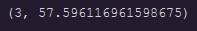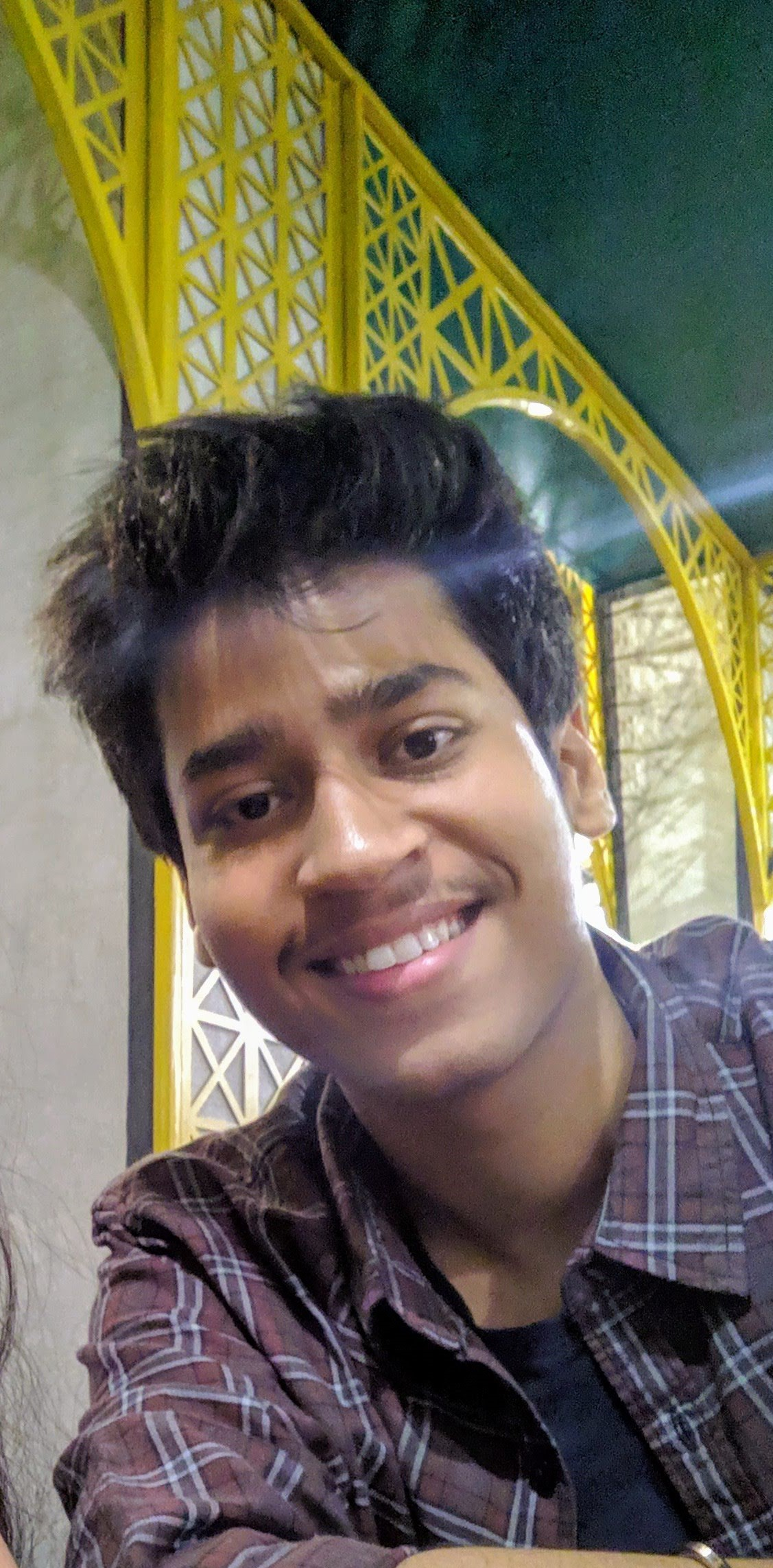## Introduction

LBPH (Local Binary Pattern Histogram) is a Face-Recognition algorithm it is used to recognize the face of a person. It is known for its performance and how it is able to recognize the face of a person from both front face and side face.

Before starting the intuition behind the LBPH algorithm, let’s first understand a little bit about the basics of Images and pixels in order to understand how images are represented before we start the content about Face-Recognition. So let”s get started understanding images and pixels.

## Images & Pixel

All images are represented in the Matrix formats, as you can see here, which are composed of rows and columns. The basic component of an image is the pixel. An image is made up of a set of pixels. Each one of these is small squares. By placing them side by side, we can form the complete image. A single pixel is considered to be the least possible information in an image. For every image, the value of pixels ranges between 0 to 255.

This image here is 32 pixels wide and 32 pixels high.

And when we multiply 32 by 32, the result is 1024, which is the total number of pixels in the image. Each pixel is composed of Three values are R, G, and B, which are the basic colours red, green, and blue. The combination of these three basic colours will create all these colours here in the image so we conclude that a single pixel has three channels, one channel for each one of the basic colours.

As now we have some understanding of images and pixels, now it will be easier to understand the intuition behind the LBPH algorithm. So let’s get started with the intuition of the LBPH algorithm

## LBPH(Local Binary Patterns Histograms)

Let’s start by analyzing a matrix that represents a piece of the image. And as you learn earlier, an image is represented in these formats. In this example, we have three rows and three columns and the total number of pixels is nine. Let’s select the central pixel here, value eight, and apply a condition. If the value is greater or equal to 8, the result is ‘1’ otherwise, if the value is less than eight, the result is zero. After applying the conditioner, the matrix will now look like this.

The basic calculation of this algorithm is to apply this condition, selecting the centre element of the matrix. Now we need to generate a binary value.Binary value = 11100010 . The algorithm will start applying the condition from the top left corner element goes up to the 1 element of the 2nd row think like it is making a circle like this.

After converting the Binary value to the decimal value we get Decimal Value = 226. It indicates that all these pixels around the central value equal to 226.

This algorithm is robust when it comes to lightning. If you put a flashlight on the image, the value of the pixels will increase. Higher the values the brighter the image and when values are lower darker the image will be. For this reason, this algorithm has good results in light and dark images because when the image becomes lighter or darker, all the pixels in the neighbourhood here will be changed. After putting the light on the image the matrix will look like this. After applying the above condition we will get the binary value the same as above i.e 11100010

Let’s consider this another image here. In order to better understand how the algorithm will recognize a person’s face.

We have the image of a face here, and what the algorithm will do is create several squares, as you can see here. And within each one of these squares, we have the representation of the previous is light. For example, this square here does not represent only one pixel but is set with multiple pixels that are three rows and four columns. Three by four is equal to twelve pixels in total in these squares here in each of these squares that are twelve pixels. And then we apply that condition to each one. Considering the central pixel.

The next step is to create a histogram, which is a concept of statistics that will count how many times each color appears in each square. This is the representation of the histogram.

For example, if the value 110 appears 50 times a bar like this will be created with this size equal to 50, if 201 appears 110 times and the other bar will be created in this histogram with this size equal to 100. Based on the comparison of the histograms, the algorithm will be able to identify the edges and also the corners of the images. For example, in this first square here, we don’t have information about the person’s face. So the histogram will be different from this other square that has the border of the face. In short, the algorithm knows which histograms represent borders and which histograms represent the person’s main features, such as the colour of the eye, the shape of the mouth, and so on.

## Now let’s start the coding part

Note:- If you are facing an error while importing the cv2 library like “No module named ‘cv2.cv2”. Then you can write code in google colab. I have written this code in Google Colab.

I will be using the yaleface dataset

### 1.Importing Libraries

```import os

import cv2

import zipfile

import numpy as np

### 2.Data Gathering

```path = "/content/drive/MyDrive/Datasets/yalefaces.zip"
zip_obj = zipfile.ZipFile(file = path,mode='r')
zip_obj.extractall('./')
zip_obj.close()```

### 3.Data Cleaning

Before giving data to the model these images are in .gif format so we need order to convert them into ndarray so we need to use the following code

`from PIL import Image`
`def get_image_data() :`
`                  paths = [os.path.join("/content/yalefaces/train",f)for f in os.listdir(path="/content/yalefaces/train")]`
```                  faces = []
ids = []```
```                  #faces will contain the px of the images
#path will contain the path of the images```
`                  for path in paths :`
```                      image = Image.open(path).convert('L')
image_np = np.array(image,'uint8')
id = int(os.path.split(path).split(".").replace("subject"," "))
ids.append(id)
faces.append(image_np)

return np.array(ids),faces```
`ids , faces = get_image_data()`

### 4.Model Training

```lbph_classifier = cv2.face.LBPHFaceRecognizer_create()
lbph_classifier.train(faces,ids)

#Below line will store the histograms for each one of the iamges
lbph_classifier.write('lbph_classifier.yml')```

### 5.Recognizing Faces

```lbph_face_classifier = cv2.face.LBPHFaceRecognizer_create()

#Now we will check the performance of model

test_image = "/content/yalefaces/test/subject03.leftlight.gif"
image = Image.open(test_image).convert('L')
image_np = np.array(image,'uint8')

#Before giving the image to the model lets check it first
cv2_imshow(image_np)
predictions = lbph_face_classifier.predict(image_np)
print(predictions)

expected_output = int(os.path.split(test_image).split('.').replace("subject"," "))
print(expected_output)
3<-That's our output```

This is the image we will be testingThe First parameter gives the face is detected, 2nd the parameter gives the confidence. This is the output we get after from print(predictions)
`cv2.putText(image_np, 'Pred.' +str(predictions),(10,30),cv2.FONT_HERSHEY_COMPLEX_SMALL,1,(0,255,0))`
```cv2.putText(image_np, 'Expec.' +str(expected_output),(10,50),cv2.FONT_HERSHEY_COMPLEX_SMALL,1,(0,255,0)
)
cv2_imshow(image_np)```

Final Result

## Conclusion:-

• What is the LBPH algorithm
• How LBPH algorithm recognize face and do calculations
• Understand code how to recognize face using LBPH algorithm

If you like my article give it like!

Something not mentioned or want to share your thoughts? Feel free to comment below And I’ll get back to you.